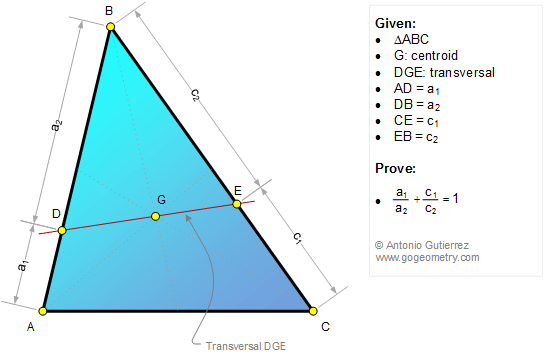# Geometry Problem 1179: Triangle, Centroid, Transversal, Sum of Ratios, Sides

< PREVIOUS PROBLEM  |  NEXT PROBLEM >

In a triangle ABC, G is the centroid and line DGE is a transversal, D on AB and E on BC (see the figure). If AD = a1, DB = a2, CE = c1, and EB = c2, prove that.